NEET  >  Test: Ionic Equation

# Test: Ionic Equation

Test Description

## 20 Questions MCQ Test Chemistry Class 11 | Test: Ionic Equation

Test: Ionic Equation for NEET 2023 is part of Chemistry Class 11 preparation. The Test: Ionic Equation questions and answers have been prepared according to the NEET exam syllabus.The Test: Ionic Equation MCQs are made for NEET 2023 Exam. Find important definitions, questions, notes, meanings, examples, exercises, MCQs and online tests for Test: Ionic Equation below.
Solutions of Test: Ionic Equation questions in English are available as part of our Chemistry Class 11 for NEET & Test: Ionic Equation solutions in Hindi for Chemistry Class 11 course. Download more important topics, notes, lectures and mock test series for NEET Exam by signing up for free. Attempt Test: Ionic Equation | 20 questions in 30 minutes | Mock test for NEET preparation | Free important questions MCQ to study Chemistry Class 11 for NEET Exam | Download free PDF with solutions
 1 Crore+ students have signed up on EduRev. Have you?
Test: Ionic Equation - Question 1

### The degree of dissociation of water in a 0.1 M aqueous solution of HC1 at a cedain temperature t°C is 3.6 x 10-15. The temperature t must be :

Detailed Solution for Test: Ionic Equation - Question 1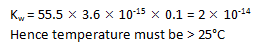Test: Ionic Equation - Question 2

### Which one is the correct expression below for the solution containing 'n' number of weak acids?

Detailed Solution for Test: Ionic Equation - Question 2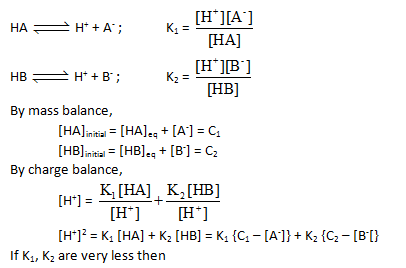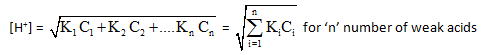Test: Ionic Equation - Question 3

### The pH of glycine at the first half equivalence point is 2.34 and that at second half equivalence paint is 9.60. At the equivalence point (The first inflection point) The pH is :

Detailed Solution for Test: Ionic Equation - Question 3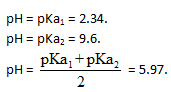Test: Ionic Equation - Question 4

The pK, of a weak acid if titration progress is monitored as followsDetailed Solution for Test: Ionic Equation - Question 4

In the horizontal part of the curve, the solution is acting as buffer. At the inflection point, [salt]=[acid]. Hence, pH = pK​= 3.88.

Test: Ionic Equation - Question 5

A 1.458 g of Mg reacts with 80.0 ml of a HCI solution whose pH is -0.477. The change in pH after all Mg has reacted. (Assume constant volume. Mg = 24.3 g/mol.)(log 3 = 0.477)

Detailed Solution for Test: Ionic Equation - Question 5

Mg (aq) + 2HCl(aq) → MgCl2 (aq) + H

1.458/24.3

Millimoles of HCl = 3 x 80 = 240 mM
Moles of HCL after reaction = 240 - 60 x

New Molarity = 120/80 = 1.5 M

pH = -log[H+] = -log 1.5 = -0.176

change is pH = -0.176 - (-0.477) = 0.3

Test: Ionic Equation - Question 6

Equal volume of two solution having pH = 2 and pH = 10 are mixed together at 90°C. Then pH of resulting solution is : (Take Kw at 90°C = 10-12)

Detailed Solution for Test: Ionic Equation - Question 6

kW = 10-12
Therefore range of pH scale. 0 to -log10-12
= 0 to 12
Since we have a strong acid and strong base mixture, the resultant solution will be neutral. So, the solution will have pH = 6

Test: Ionic Equation - Question 7

Find the ApH(initial pH -final pH) when 100 ml 0.01 M HCI is added in a solution containig 0.1 m moles of NaHCO3 solution of negligible volume ( Kai =10-7, Ka, =10-11 for H2CO3) :

Detailed Solution for Test: Ionic Equation - Question 7

pH of NaHCO3 solution = 9

Now H+ + HCO3 → HCO3

∴ no. of m mole of HCl remaining = 1 - 0.1= 0.9 m mole

∴ pH = -log (9 x 10-3) = -2 log 3 + 3

Test: Ionic Equation - Question 8

The ionization constant of benzoic acid is 6.46 x 10-5 and lc for silver benzoate is 2.5 x 10-13. How many times silver benzoate more soluble in a buffer of pH = 3.19 as compared to its solubility in pure water?

Detailed Solution for Test: Ionic Equation - Question 8

The correct answer is Option A.

Ka of PhCOOAg= 6.45×10-5
Ksp of PhCOOAg= 2.5×10-13
pH of buffer = 3.19
∴[H] =Antilog(−3.19)
=Antilog(−3−0.19+1−1)
=Antilog(4.81)
=6.45×10-4 M
Let Sbuffer(solubility of salt in a buffer)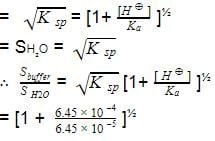=[1+10]1/2
=(11)1/2
=3.317
Thus, silver benzoate is
3.317 times more soluble at lower
pH than in water.

Test: Ionic Equation - Question 9

30 ml of 0.06 M solution of the protonated form of an anion acid methonine (H2A+) is treated with 0.09 M NaOH. Calculate pH after addition of 20 ml of base. pKa, = 2.28 and pKa2 = 9.2.

Detailed Solution for Test: Ionic Equation - Question 9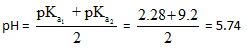Test: Ionic Equation - Question 10

A certain acid-base indicator is red in acid solution and blue in basic solution 75% of the indicator is present in the solution in its blue form at pH = 5. Calculate the pH at which the indicator shows 90% red form?

Detailed Solution for Test: Ionic Equation - Question 10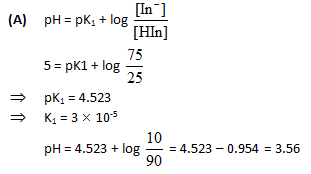Test: Ionic Equation - Question 11

Ionisation constant of HA (weak acid) and BOH (weak base) are 3.0 x 10-7 each at 298K. The percent degree of hydrolysis of BA at the dilution of 10L is :-

Detailed Solution for Test: Ionic Equation - Question 11

B+    +   A-   +  H2O  ⇋  BOH   +   HA
1-α        1-α                     α           α
α/1-α = √kHydrolysis = √kWater/kacid
kbase
= √(10-14)/(3×10-7)2
= ⅓
Or α = 0.25
Therefore, %age dissociation = 25%

Test: Ionic Equation - Question 12

Which of the following concentrations of NH4+ will be sufficient to prevent the precipitation of Mg(OH)2 from a solution which is 0.01 M MgCl2 and 0.1 M NH3 (aq).

Given that : K5 of Mg (OH)2 = 2.5 x 10-11 and Kb for NH3(aq) = 2 x 10-6.

Detailed Solution for Test: Ionic Equation - Question 12

The expression for the solubility product of magnesium hydroxide is Ksp
[Mg2+][OH-]2
Thus 2.5×10-11 = 0.01 × [OH-]2
[OH-] = 5×10-5
The expression for dissociation constant of base is Kb = [NH4+][OH-]/[NH3]
Thus = 2×10-5 = [NH4+](5×10-5)/0.1
Hence, [NH4+] = 0.04 M

Test: Ionic Equation - Question 13

Calculate the molar solubility of zinc tetrathiocyanato-N-mercurate (II) if its Ksp = 2.2 x 10-7.

Detailed Solution for Test: Ionic Equation - Question 13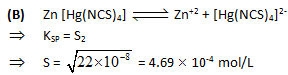Test: Ionic Equation - Question 14

An acid-base indicator which is a weak acid has a pKa value = 5.45. At what cocentration ratio of sodium acetate to acetic acid would the indicator show a colour half-way between those of its acid and conjugate base forms? pKa of acetic acid = 4.75. [log 2 = 0.3]

Detailed Solution for Test: Ionic Equation - Question 14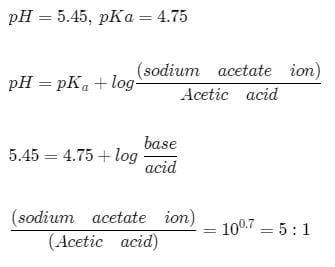Test: Ionic Equation - Question 15

The indicator constant of phenolphthalein is approximately 10-10. A solution is prepared by adding 100.01 c.c. of 0.01 N sodium hydroxide to 100.00 c.c. of 0.01N hydrochloric acid. If a few drops of phenolphthalein are now added, what fraction of the indicator is converted to its coloured form?

Detailed Solution for Test: Ionic Equation - Question 15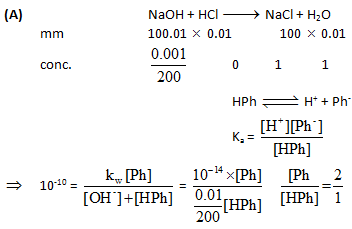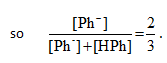Test: Ionic Equation - Question 16

A certain mixture of HCI and CH3 - COOH is 0.1 M in each of the acids. 20 ml of this solution is titrated against 0.1M NaOH. By how many units does the pH change from the start to the stage when the HCI is almpst completely neutralised? Ka for acetic acid = 1.8 x 10-6.

Detailed Solution for Test: Ionic Equation - Question 16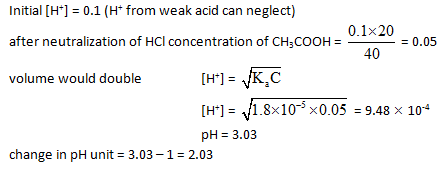Test: Ionic Equation - Question 17

A buffer solution is made by mixing a weak acid HA (Ka = 10-6) with its salt NaA in equal amounts. What should be the amount of acid or salt that should be added to make 90 ml of buffer solution of buffer capacity. 0.1 ?

Detailed Solution for Test: Ionic Equation - Question 17

For buffer capacity of 0.1 we should have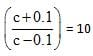where is concentration of weak acid or salt in the buffer solution

So, c+0.1 = 10c-1,

so 9c = 1.1 or c= 1.1/9

So, moles required for 90 solution =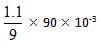moles = 11 m moles

Test: Ionic Equation - Question 18

A sample of water has a hardness expressed as 80 ppm of Ca2+ . This sample is passed through an ion exchange column and the Ca2+ is replaced by H+. What is the pH of the water after it has been so treated? [Atomic mass of Ca = 40]

Detailed Solution for Test: Ionic Equation - Question 18

106 ml water contains 80gm of ca2+ = 80/40 moles = 2 moles of ca2+ = 2 x 2 moles of H+ ions so
103 ml of H2O will have = 4 x 10-3 moles of h+ ions
so pH = 3 - log 4 = 3-0.6 = 2.4

Test: Ionic Equation - Question 19

Statement-1 : solubility of BaSO4 in 0.1 M Na2SO4 is 10-9 M hence its K8 is 10-18.

Statement-2 : because for BaSO4 Ksp = (s)2 [symbols have their usual meanings].

Detailed Solution for Test: Ionic Equation - Question 19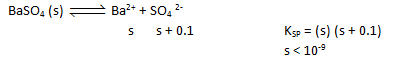Test: Ionic Equation - Question 20

Statement-1 : It is difficult to distinguish the strengths of the strong acids such as HCI, H2SO4, HNO3,HBr, HI or HCIO4 in dilute aqueous solutions.

Statement-2 : In dilute aqueous solution all strong acids donate a proton to water and are essentially. 100% ionised to produce a solution containing H30+ ions plus the anions of strong acid .

Detailed Solution for Test: Ionic Equation - Question 20

All are strong (α = 1) (leavelling effect).

## Chemistry Class 11

204 videos|331 docs|229 tests
 Use Code STAYHOME200 and get INR 200 additional OFF Use Coupon Code
Information about Test: Ionic Equation Page
In this test you can find the Exam questions for Test: Ionic Equation solved & explained in the simplest way possible. Besides giving Questions and answers for Test: Ionic Equation, EduRev gives you an ample number of Online tests for practice

## Chemistry Class 11

204 videos|331 docs|229 tests

### How to Prepare for NEET

Read our guide to prepare for NEET which is created by Toppers & the best Teachers